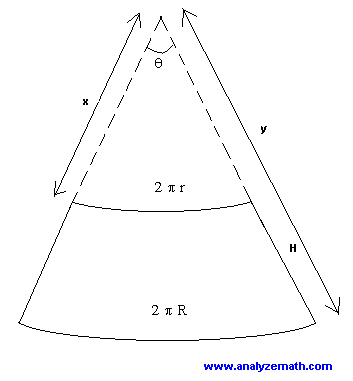# Surface Area and Volume of Frustum - Geometry Calculator

Online calculator to calculate the surface area and the volume of a Frustum given its radius R at the base, its radius r at the top and its height h.(see figure below).This calculator also calculates the parameters needed for the construction of a frustrum given R, r and h. If you cut the frustrum along the along the slanted height H, you obtain a shape similar to the one on the right below.If you now prolong the sides of the figure on the right above, you obtain the sector shown below.To construct the frustum, you need to find x, y and the central angle t. The formulas for these perimeters may be found in
How to construct a frustum?. Here you may use the calculator below to calculate these parameters.

How to use the calculator

Enter radius r (radius at top), radius R (radius at bottom) with r < R and height h of the frustum as positive real numbers and press "calculate". The outputs are the lateral surface area, the total surface area (including the base and bottom), the volume of the frustum and parameters x, y and angle t for the construction of a frustrum given r, R and h.

 r = R = h = Lateral Area = Total Area = Volume = x = y = t = degrees
More Online Geometry Calculators and Solvers.